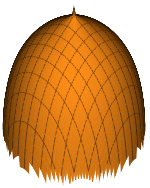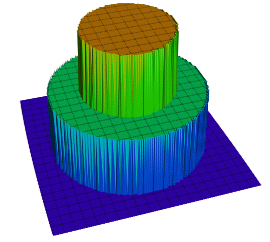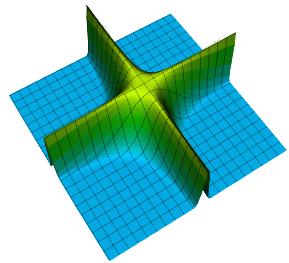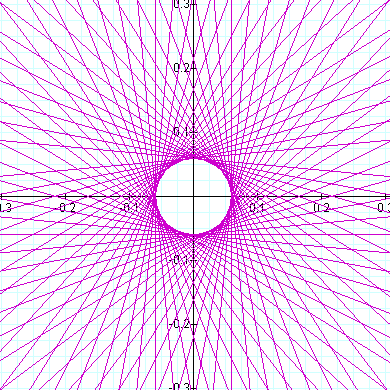﻿ SuperCalculator - 3D Graphing Calculator

#### Plot of 3D Functions

This procedure is similar to X-Y-Z Grid Plot except that instead of reading the grid values from the data matrix, it will produce its own grid from a function and intervals supplied by the user.

All controls except F(X,Y) and Plot Frequency will work in exactly the same way as they do in 3D Plots procedure. The F(X,Y) field is used for entering a formula in terms of the variables X and Y. No other variable names can be used. Either type the formula directly into the text box or double-click on it to open the Formula Editor dialogue. Plot of Functions.

The Plot Frequency control will regulate the resolution of plots. The highest resolution is obtained when this field is set to 1. In this case the function will be evaluated 50 times for each axis giving a total of 2,500 function evaluations. The lowest resolution is 10, which means that the maximum grid size 50 will be divided by 10, giving 5 polygons (or 5 bars or 5 points) for each axis.

Since computing the grid elements from the supplied function may be a time consuming process, it will not be repeated whenever a graph property is changed. For instance the grid will not be recomputed if changes are made in the main title, sub title, X, Y and Z axis labels, minimum, maximum and interval values of the Z axis, type, rotation, grid and colours fields. If changes are made in one of minimum, maximum X or Y-axis values, the definition of function or the Plot Frequency, then the whole grid will be recomputed.

#### All Available Functions

##### Operators

Subtraction operator

Multiplication operator

Division operator

Exponent (Power) operator

Base operator

##### Functions

Square Root of a value or expression.

sine of a value or expression

cosine of a value or expression

tangent of a value or expression

inverse sine (arcsine) of a value or expression

inverse cosine (arccos) of a value or expression

inverse tangent (arctangent) of a value or expression

Hyperbolic sine (sinh) of a value or expression

Hyperbolic cosine (cosh) of a value or expression

Hyperbolic tangent (tanh) of a value or expression

e (the Euler Constant) raised to the power of a value or expression

The natural logarithm of a value or expression

The base-10 logarithm of a value or expressioN

Returns the largest (closest to positive infinity) value that is not greater than the argument and is equal to a mathematical integer.

Returns the smallest (closest to negative infinity) value that is not less than the argument and is equal to a mathematical integer.

Round to the nearest integer. Examples: round(-2.5) = -2, round(-0.1) = 0, round(0.1) = 0, round(2.5) = 3

Sign (+1 or -1) of a value or expression

Absolute value (distance from zero) of a value or expression

## Water Ripple 3D Equation

sin(10(x^2+y^2))/10## Paper Plane 3D Equation

sign(x) * atan(x*80)/6 * sign(-y-x+1) * sign(-y+x+1)*5 - 1.01## Mosque Dome 3D Function

0.7/log(x^2+y^2)+.6## Top Hat 3D Equation

(sign(0.2-(x^2+y^2)) + sign(0.2-(x^2/3+y^2/3)))/3-1## Paper Plane 3D Equation

sign(x) * atan(x*80)/6 * sign(-y-x+1) * sign(-y+x+1)*5 - 1.01## Intersecting Fences

0.75/exp((x*5)^2*(y*5)^2)## Interlock Equation

(3x/2)^4 + (3x)^4 + z^4 = 1, (3x)^4 + y^4 + (3z/2)^4 = 1, x^4 + (3y/2)^4 + (3z)^4 = 1## Scatered Equation

Cos(x) + Cos(y) + Cos(z) + Sin(x) + Sin(y) + Sin(z) = 2.2## Unamed Equation

r = Tan(17 theta) + Cot(17 theta)